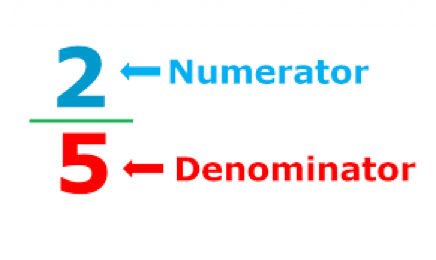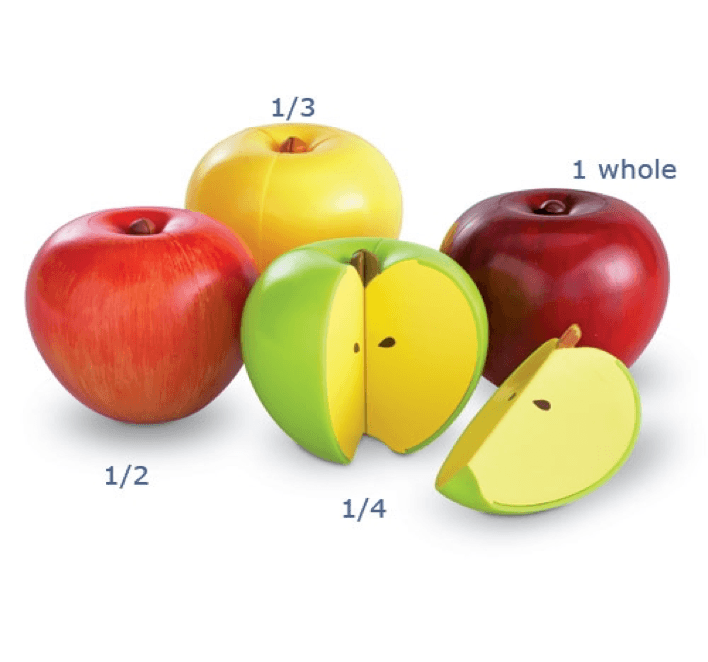# How to Teach Fractions: All You Need to Know

TeachingLearning fractions can be hard for many children because it goes beyond basic simple arithmetic calculations. To understand the concept, kids need to practice with examples every day before they can master fractions. This article provides tips on how to learn fractions in a fun and engaging way.

## What is a fraction?

A fraction is a number that indicates a part of a whole. One can write a fraction using two numbers separated by a line instead of a division symbol.

The number above the line is a numerator and represents the number of parts you have. Similarly, the number below the line is called a denominator and represents the total number of parts in the whole area or object.

A simple fraction shows one part that has been partitioned into equal parts. A proper fraction is the one where a numerator is strictly less than a denominator. An improper fraction has a numerator that is greater than or equal to its denominator.

When teaching fractions, you can show kids that 2/5 means that 2 is divided by 5. Each piece that we divide the whole into is called a part, and each part has an equal value. The number on top tells us how many parts we have.## How to teach fractions to a child?

At what grade do you learn fractions? Kids meet fractions as early as in their first grade. At this stage, you can draw circles or squares with different colors and then divide those shapes into parts. Such pictures will be among the fun ways to teach fractions.

While learning fractions for beginners, you can use colors. For example, you can divide a circle into 2 equal parts and apply blue color for one part and red color for the other part of the circle.

Such an approach will help kids understand that half of the circle is blue and half of it is red, which will let children understand fractions without using any numbers or symbols.

Once children master this concept, you can introduce multiplication. For example, start with simple examples like 1/2 × 1/3 = 1/6 if you are not sure how to teach multiplying fractions.

Math for Kids

Is Your Child Struggling With Math?
1:1 Online Math Tutoring### The Nominator

A nominator is a number that indicates how many parts of a whole you have. In other words, a nominator is simply a number that represents the value of the fraction.

A nominator is the first part of a fraction. The other part is called a denominator, which is the bottom number in a fraction.

The purpose of a nominator in fractions is to tell you how many parts you have or what number to count up to. The following is an example of how to teach simplifying fractions:

2/3 means 2 out of 3 parts. So, if you were to cut a cake into 3 equal slices, you would eat 2 of them. Fraction explained, right?

1/2 means 1 out of 2 parts. If you cut that same cake into 2 slices, you would eat 1 slice and leave 1 slice for someone else to eat.

Another example is the following: if you have a pizza and slice it into eight pieces, each piece would be one-eighth of the pizza. You could also say that each piece is “one over eight” or “one/eight” of the whole pizza.### The Numerator

A numerator is a term used in mathematics that refers to the number above the line in a common fraction. You can think of a numerator as an indicator representing the number of parts you want to take from the whole.

For example, in the fraction 5/8, “5” is the numerator, while the number below the line (8) is called the denominator.

A numerator represents many equal parts, indicating how many of those parts are taken or considered. In the fraction 7/10, for instance, seven parts are taken out of ten equal parts.

### Fraction Notation

Fraction notation is a form of writing simple fractions, ratios, and other numbers. It is represented by two digits or numbers separated by a line and placed over each other. In other words, fractional notation is a way to express ratios using the division sign and slashes.

Fractions are usually written using the slash symbol (/) but can also be presented as a division equation with a division sign (÷).

The examples are 3/4, 12/16, 1/3, etc. If you write 3/4, it means that there are three-quarters of something. This thing can be any object or concept. 1/2 is one half, 1/3 is one-third, and so on.

### Fractions in Everyday LifeThere are numerous cases in everyday life where we apply fractions. They not only show up in math class but also can help measure things such as distance or time. For example, when measuring distance, we use fractions of a mile or a kilometer.

When you go to the gas station, you will see pumps that measure gasoline by fractions of gallons. Gallons are divided into halves, quarters, and halves or quarter’s eighths. More importantly, such division makes it easier to fill up a vehicle’s gas tank because some tanks only hold certain amounts of fuel.

Fractions are useful in cooking recipes. We use them when we are measuring ingredients while cooking.  Recipes require you to cut, halve, or double ingredients.

In addition, measurements of liquids may require you to use fractions. Fractions also show up on measuring tapes and rulers to measure parts of an inch and parts of a foot accurately.

Another application of fractions is measuring time. For example, if you have three-quarters of an hour to complete an exam, you will have 45 minutes to complete the exam since there are 60 minutes in one hour.

## Conclusion

The process of learning fractions does not have to be tedious. Numerous useful tips can help make math lessons more interesting and engaging for students. A truly effective math tutor will know how to explain fractions to convey the mathematical concepts in a creative way that children love.

Math Catch Up Program• Learn Math Simple - Fast - Effective
• Overcome math obstacles and reach new heights with Brighterly.

Simple - Fast - Effective
Overcome math obstacles and reach new heights with Brighterly.